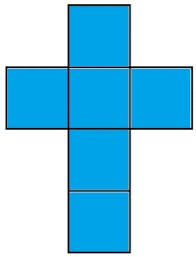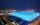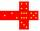# Cube surfce2volume

Calculate the volume of the cube if its surface is 150 cm2.

V =  125 cm3

### Step-by-step explanation:Did you find an error or inaccuracy? Feel free to write us. Thank you!Tips to related online calculators
Tip: Our volume units converter will help you with the conversion of volume units.

## Related math problems and questions:

• The cubeThe cube has a surface area of 216 dm2. Calculate: a) the content of one wall, b) edge length, c) cube volume.
• Volume and areaWhat is the volume of a cube which has an area of 361 cm2?
• Area to volumeIf the surface area of a cube is 486, find its volume.
• The cubeThe cube has a surface area of 486 m ^ 2. Calculate its volume.
• TerezaThe cube has an area of base 256 mm2. Calculate the edge length, volume, and area of its surface.
• The cubeThe cube has a surface of 600 cm2. What is its volume?
• Cube surface and volumeThe surface of the cube is 500 cm2, how much cm3 will be its volume?
• Cube 6Volume of the cube is 216 cm3, calculate its surface area.
• Area of a cubeCalculate the surface area of a cube if its volume is equal to 729 cubic meters.
• Cube surface and volumeFind the surface of the cube with a volume of 27 dm3.
• Cube 6The surface area of one wall cube is 1600 cm square. How many liters of water can fit into the cube?
• The cubeThe surface of the cube is 150 square centimeters. Calculate: a- the content of its walls b - the length of its edges
• Cube and sphereCube with the surface area 150 cm2 is described sphere. What is sphere surface?
• Cube V2SThe volume of the cube is 27 dm cubic. Calculate the surface of the cube.
• Cube 7Calculate the volume of a cube, whose sum of the lengths of all edges is 276 cm.
• Three cubesTwo cube-shaped boxes with edges a = 70 cm; b = 90 cm must be replaced by one cube-shaped box. What will be its edge?
• CubeThe sum of lengths of cube edges is 57 cm. What is its surface and volume?